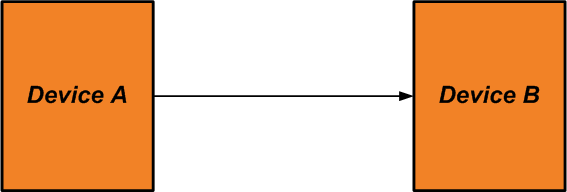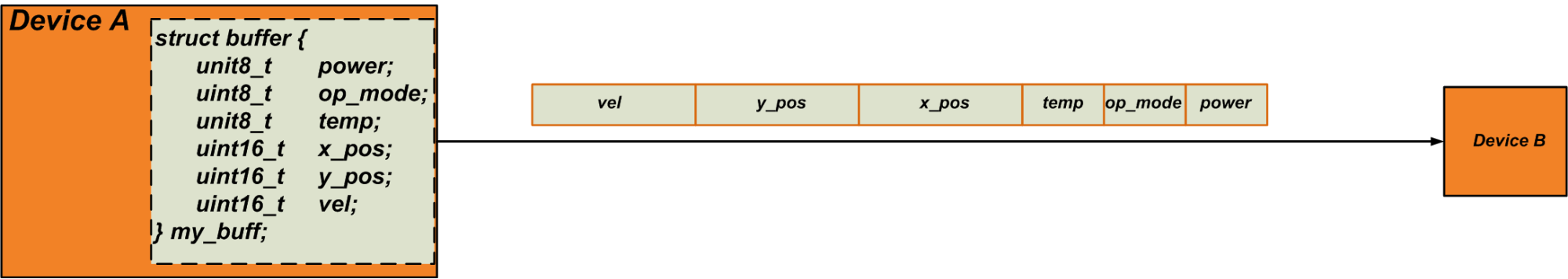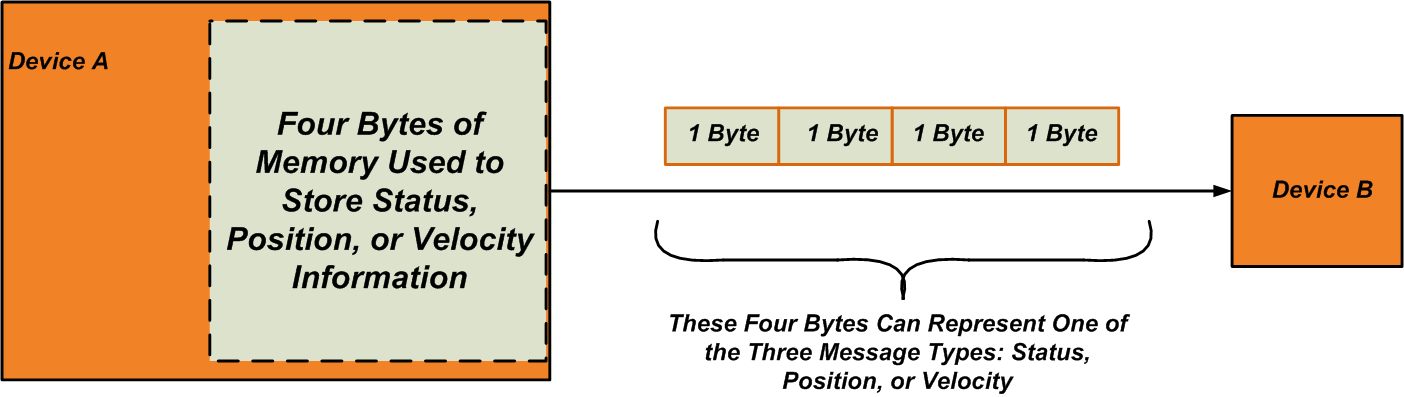Technical Article

# Learn the Embedded C Programming Language: Understanding the Union Data Object

May 21, 2019 by Steve Arar

## Learn about data objects called unions in embedded C language.

Learn about data objects called unions in embedded C language.

### The Difference Between Structure and Union in Embedded C

In a previous article of this series, we discussed that structures in embedded C allow us to group variables of different data types and deal with them as a single data object.

In addition to structures, the C language supports another data construct, called a union, that can group different data types as a single data object. This article will provide some basic information about unions. We’ll first take a look at an introductory example of declaring a union, then we’ll examine an important application of this data object.

### Introductory Example

Declaring a union is much like declaring a structure. We only need to replace the keyword “struct” with “union”. Consider the following example code:

union test {
uint8_t	    c;
uint32_t    i;
};

This specifies a template that has two members: “c”, which takes one byte, and “i”, which occupies four bytes.

Now, we can create a variable of this union template:

union test u1;

Using the member operator (.), we can access the members of the “u1” union. For example, the following code assigns 10 to the second member of the above union and copies the value of “c” to the “m” variable (which must be of type uint8_t).

u1.i=10;
m=u1.c;

How much memory space will be allocated to store the “u1” variable? Whereas the size of a structure is at least as large as the sum of the sizes of its members, the size of a union is equal to the size of its largest variable. The memory space allocated to a union will be shared among all the union members. In the above example, the size of “u1” is equal to the size of uint32_t, i.e., four bytes. This memory space is shared between “i” and “c”. Hence, assigning a value to one of these two members will change the value of the other member.

You may be wondering, "What’s the point of using the same memory space to store multiple variables? Is there any application for this feature?" We’ll explore this issue in the next section.

### Do We Need Shared Memory Space?

Let’s look at an example where a union can be a useful data object. Assume that, as shown in Figure 1 below, there are two devices in your system that need to communicate with each other.##### Figure 1

“Device A” should send status, velocity, and position information to “Device B”. The status information consists of three variables that indicate the battery charge, the mode of operation, and the ambient temperature. The position is represented by two variables that show the x- and y-axis positions. Finally, the velocity is represented by a single variable. Assume that the size of these variables is as shown in the following table.

 Variable Name Size (Byte) Explanation power 1 Battery Charge op_mode 1 Mode of Operation temp 1 Temperature x_pos 2 X Position y_pos 2 Y Position vel 2 Velocity

If “Device B” constantly needs to have every piece of this information, we can store all of these variables in a structure and send the structure to “Device B”. The structure size will be at least as large as the sum of the size of these variables, i.e., nine bytes.

Thus, every time that “Device A” talks to “Device B”, it needs to transfer a 9-byte data frame through the communication link between the two devices. Figure 2 depicts the structure that “Device A” uses to store the variables and the data frame that needs to go through the communication link.##### Figure 2

However, let’s consider a different scenario where we only occasionally need to send the status information. Also, suppose that it’s not necessary to have both position and velocity information at a given time. In other words, sometimes we only send position, sometimes we only send velocity, and sometimes we send only status information. In this situation, it doesn’t seem like a good idea to store the information in a nine-byte structure and transfer it through the communication link.

Status information can be represented by only three bytes; for position and velocity, we need only four and two bytes, respectively. Therefore, the maximum number of bytes that “Device A” needs to send in one transfer is four, and consequently, we need only four bytes of memory to store this information. This four-byte memory space will be shared among our three message types (see Figure 3).

Additionally, note that the length of the data frame passed through the communication link is reduced from nine bytes to four bytes.##### Figure 3

To summarize, if our program has variables that are mutually exclusive, we can store them in a shared area of memory to preserve valuable memory space. This can be important, especially in the context of memory-constrained embedded systems. In such cases, we can use unions to create the required shared memory space.

The above example shows that using a union to handle mutually exclusive variables can also help us conserve communication bandwidth. Conserving communication bandwidth is sometimes even more important than conserving memory.

### Using Unions for Message Packets

Let’s see how we can use a union for storing the variables of the above example. We had three different message types: status, position, and velocity. We can create a structure for the variables of the status and position messages (so that the variables of these messages are grouped and manipulated as a single data object).

The following structures serve this purpose:

struct {
uint8_t 	power;
unit8_t	        op_mode;
uint8_t	        temp;
} status;

struct {
uint16_t 	x_pos;
unit16_t	y_pos;
} position;

Now, we can put these structures along with the “vel” variable in a union:

union {
struct {
uint8_t 	power;
unit8_t	        op_mode;
uint8_t	        temp;
} status;

struct {
uint16_t 	x_pos;
unit16_t	y_pos;
} position;

uint16_t	vel;

} msg_union;

The above code specifies a union template and creates a variable of this template (named “msg_union”). Inside this union, there are two structures (“status” and “position”) and a two-byte variable (“vel”). The size of this union will be equal to the size of its largest member, namely, the “position” structure, which occupies four bytes of memory. This memory space is shared among “status”, “position”, and “vel” variables.

### How to Keep Track of the Union Active Member

We can use the shared memory space of the above union to store our variables; however, there remains one question: How should the receiver determine which type of message has been sent? The receiver needs to recognize the message type to be able to successfully interpret the received information. For example, if we send a “position” message, all four bytes of the received data are important, but for a “velocity” message, only two of the received bytes should be used.

To solve this issue, we need to associate our union with another variable, say “msg_type”, that indicates the message type (or the union member that was last written to). A union paired with a discrete value that indicates the active member of the union is called a “discriminated union” or a “tagged union”.

Regarding the data type for the “msg_type” variable, we can use the enumeration data type of the C language to create symbolic constants. However, we’ll use a character to specify the message type, just to keep things as simple as possible:

struct {
uint8_t	msg_type;
union {
struct {
uint8_t 	power;
unit8_t	op_mode;
uint8_t	temp;
} status;

struct {
uint16_t 	x_pos;
unit16_t	y_pos;
} position;

uint16_t	vel;

} msg_union;
} message;

We can consider three possible values for the “msg_type” variable: ‘s’ for a “status” message, ‘p’ for a “position” message, and ‘v’ for a “velocity” message. Now, we can send the “message” structure to “Device B” and use the value of the “msg_type” variable as an indicator of the message type. For example, if the value of the received “msg_type” is ‘p’, “Device B” will know that the shared memory space contains two 2-byte variables.

Note that we’ll have to add another byte to the data frame sent through the communication link because we need to transfer the “msg_type” variable. Also note that, with this solution, the receiver doesn’t need to know ahead of time what kind of message is coming in.

### The Alternative Solution: Dynamic Memory Allocation

We saw that unions allow us to declare a shared memory area to conserve both memory space and communication bandwidth. There is, however, another way to store mutually exclusive variables such as those in the above example. This second solution uses dynamic memory allocation to store the variables of each message type.

Again, we’ll need to have a variable “msg_type” to specify the message type at both the transmitter and the receiver end of the communication link. For example, if “Device A” needs to send a position message, it will set “msg_type” to ‘p’ and allocate four bytes of memory space to store “x_pos” and “y_pos” variables. The receiver will check the value of “msg_type” and, depending on its value, create the appropriate memory space for storing and interpreting the incoming data frame.

Use of dynamic memory can be more efficient in terms of memory usage because we are allocating just enough space for each message type. This was not the case with the union-based solution. There, we had four bytes of shared memory to store all three message types, though the “status” and “velocity” messages required only three and two bytes, respectively. However, dynamic memory allocation can be slower, and the programmer needs to include code that frees the allocated memory. That’s why programmers usually prefer to use the union-based solution.

### Next Up: Applications of Unions

It seems that the original purpose of unions was creating a shared memory area for mutually exclusive variables. However, unions have also been widely used for extracting smaller parts of data from a larger data object.

The next article in this series will focus on this application of unions, which can be particularly important in embedded applications.•Jakub Standarski June 22, 2019